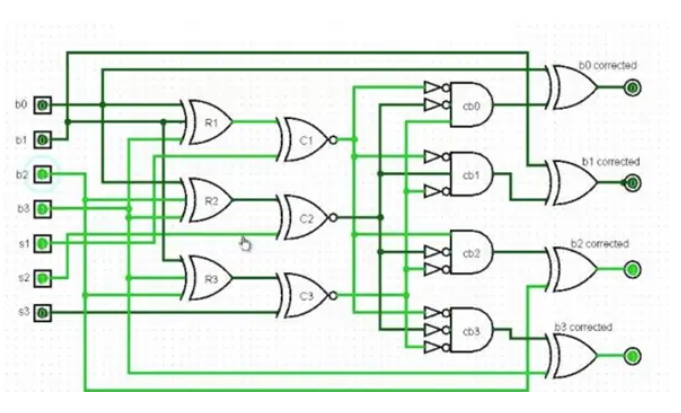# Is someone able to see my misstake?ClassicListThreaded4 messagesOpen this post in threaded view
|

## Is someone able to see my misstake?

 This post was updated on .I should represent that with the simulator. For this I have created myself xor, xnor, not and and. Therefore it is not the built-in "xor", "xnor", "not" and "and". However, they work just as well as the built-in. But I have an error, although I do not see it, because I think I created the code correctly, does anyone see it? CHIP test{ //b0=in, b1=in, b2=in, b3=in //s1=in, s2=in, s3=in         IN in;         OUT out;                 PARTS:                 //R1         Xor(a=in, b=in, out=a);         Xor(a=a, b=in, out=b);                 //R2         Xor(a=in,b=in,out=c);         Xor(a=c, b=in, out=d);                 //R3         Xor(a=in,b=in,out=e);         Xor(a=e,b=in,out=f);                 //C1         Xnor(a=b,b=in, out=h);         //C2         Xnor(a=d,b=in, out=j);         //C3         Xnor(a=f,b=in, out=l);                         Not(in=h1, out=hh);         Not(in=j1, out=jj);         Not(in=l1, out=ll);                 //cb0         And(a=hh, b=jj, out=m);         And(a=m, b=l, out=n);                 //cb1         And(a=hh, b=j, out=o);         And(a=o, b=ll, out=p);                 //cb2         And(a=h, b=jj, out=q);         And(a=q, b=l, out=r);                 //cb3         And(a=hh, b=jj, out=s);         And(a=s, b=ll, out=t);                 //b0         Xor(a=in, b=n, out=out);         //b1         Xor(a=in, b=p, out=out);         //b2         Xor(a=in, b=r, out=out);         //b3         Xor(a=in, b=t, out=out);                                 }
Open this post in threaded view
|

## Re: Is someone able to see my misstake?

 Administrator What part is this supposed to be? Your HDL says that this chip is called "test".